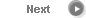#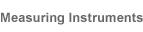# Precise Color Communication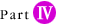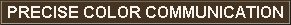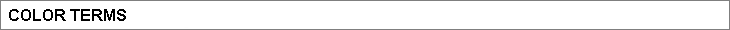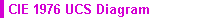The CIE 1976 UCS Diagram was defined by the CIE in 1976. It is intended to provide a perceptually more uniform color spacing for colors at approximately the same luminance. The values of u' and v' can be calculated from the tristimulus values XYZ (or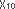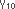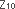) or from the chromaticity coordinates xy according to the following formulas: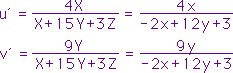where X, Y, Z: Tristimulus values (If tristimulus valuesare used, the results will be u'10 and v'10.) x, y: Chromaticity coordinates (If chromaticity coordinates x10 y10 are used, the results will be u'10 and v'10.)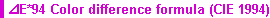This color difference formula modifies the lightness, saturation, and hue (L*C*h) of the L*a*b* color space by incorporating factors that correct for variation in perceived color difference magnitude in different areas of the CIE 1976 L*a*b* color space. This was proposed in 1994 by the technical committee of CIE.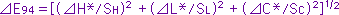where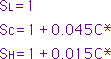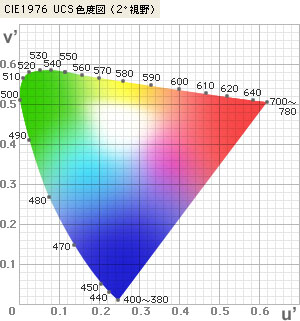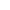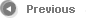8/12